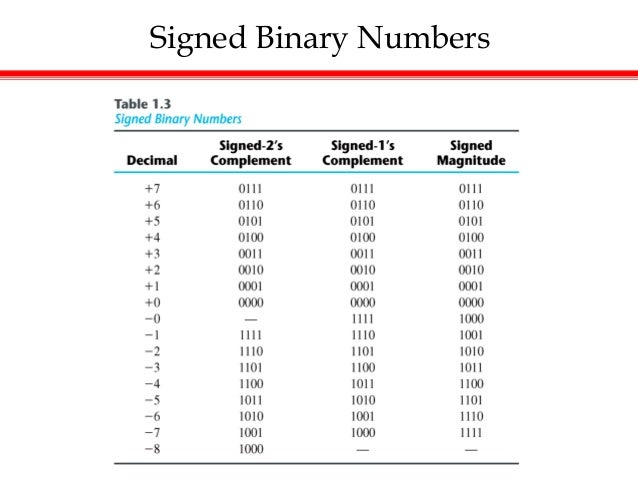# Signed Numbers To Binary OnlineBefore starting a conversion you have to choose if the numbers are signed or unsigned.

The converter allows you to;

• convert binary to decimal numbers
• convert hexadecimal to decimal numbers
• convert hexadecimal to binary numbers
• convert binary to hexadecimal numbers
• convert decimal to hexadecimal numbers
• convert decimal to binary numbers
• determine the 2's complement of a binary number
• shift right a binary number
• shift left a binary number
• rotate right a binary number
• rotate left a binary number.
• do any of the conversions above for positive and negative numbers.
• The converter is not designed to convert binary fractions.